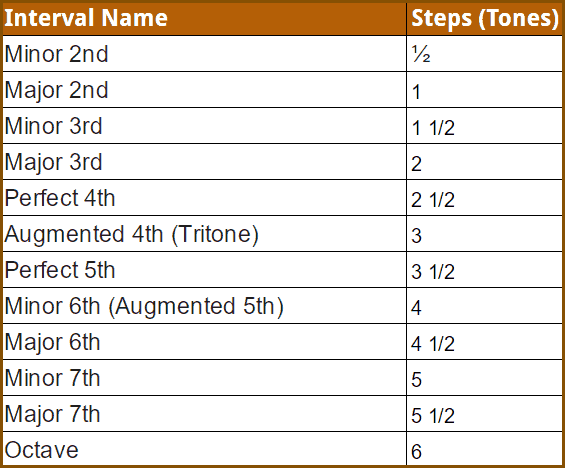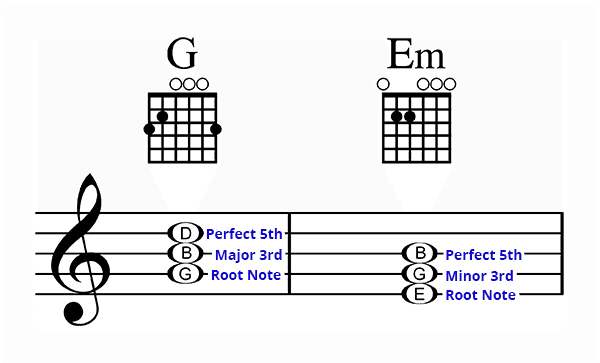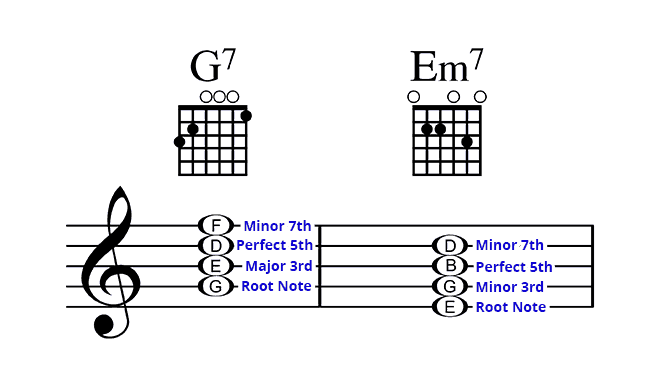# Understanding Guitar Chords – Triads And 7th Chords

June 9, 2016 in Guitar Skills# Guitar Theory Made Easy Part Two

In the previous lesson I talked about triads which are basic three note chords. If this is all brand-new to you I would encourage you to review that lesson. I'll include a link below.

Today I'm going to talk about a slightly different way of understanding chords that is very common and useful. I'll show you how chords are constructed using intervals from the root note of the chord.Intervals can be described in two different ways. One is the number of steps. For example we can say that the interval between a B note and the D note is 1 1/2 steps. Intervals also have names which make them quick and easy to refer to. In this case we would call the interval of 1 1/2 steps a minor third.

Here's a chart which shows the most common names of intervals and includes how many steps there are in each one. Today will be focusing on just a few of these intervals for creating major and minor chords.

# Distance From The Root Note

In this lesson when we talk about intervals we are describing the interval from the root of the chord. For example a major Triad consists of 3 notes: 1) the root note, 2) a note that is a major 3rd (or 2 steps) from the root note, 3) a note that is a perfect 5th (or 3 1/2 steps) from the root note.

If the root note was G, then the chord would consist of G (Root), B (Major 3rd from the root) and D (perfect 5th from the root).

This is not the only way to describe chords. In previous lessons I have also explain chords as the distance from one note to another (i.e. G to B = 2 steps; B to D = 1 1/2 steps) and as scale positions (G-B-D is 1-3-5 in the G Major scale).

Each system has value. We are just looking at it from a different point of view. They fit together like a puzzle like in the story Six Blind Students And The Elephant. What you are learning in this lesson is something I use regularly as part of my performing and composing.

The major Triad consists of a major third from the root, and then a perfect fit from the root note. This is a formula and you can follow it every time to create a major chord. The name of the chord will always be the same as the name of the root note.

For example let's take the G chord. The note G is the root. A major third from G is the note B. Then a perfect fifth from the root is the note D. The G major chord consists of the notes G, B and D.The formula for a minor Triad starts with a minor third from the root note. Then a perfect fifth from the root note. Again you can follow this formula every time and you'll end up with a minor chord.

For example will use the E minor chord. The note E is the root of the chord. Going up a minor third from E is the note G. A perfect fifth from the root is the note B. and E minor chord consist of the notes E, G and B.

# Understanding 7th Chords - G7

Now let's extend the triad a little bit. Were going to add the interval of a minor seventh to the chord to create a seventh chord, also known as a dominant seventh chord.

So to that G Triad were going to add the F note which is a minor 7th interval from the root note. And easier way to find the minor 7th interval then counting up from the root is to count back one whole step. In this case going back one step from G gives us F.# Understanding Minor 7th Chords - Em7

We'll do the same with the E minor chord. Let's add another note that is a minor 7th interval from the root note E. Now we have the following notes: E, G, B, and D.

The formula for a minor 7th chord is the root note, a minor 3rd, the perfect 5th and a minor 7th. If you follow this formula you'll always get a minor 7th chord with the name of the root note.

#### Recommended Resources:

In this lesson I explain more about the basic chords called triads and how they relate to the guitar neck.

Tell Us What You Think - Please Comment Below!

We would love to hear your comments and questions. What specific things are you struggling with while learning guitar?